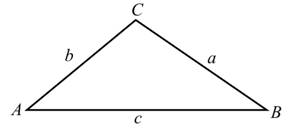Chapter 14.3, Problem 6E

Chapter
Section
Textbook Problem

# Solve each triangle using the labels as shown in Illustration 1 (round lengths of sides to three significant digits and angles to the nearest tenth of a degree):ILLUSTRATION 1 B = 24.7 ° , C = 136.1 °   , a = 342  m

To determine

To calculate: The lengths of each side and each angle of the illustrated triangle below where B=24.7°,C=136.1°,a=342m,Explanation

Given Information:

The provided sides are, B=24.7°,C=136.1°,a=342m and figure is,

Formula Used:

According to law of Sines,

asinA=bsinB=csinC

According to angle sum property of triangle,

A+B+C=180°

Calculation:

Consider the figure below,

By the law of Sines,

asinA=bsinB=csinC

By using the above formula,

342sinA=bsin24.7=csin136.1

According to angle sum property of triangle,

A+B+C=180°

By using above formula,

A+24

### Still sussing out bartleby?

Check out a sample textbook solution.

See a sample solution

#### The Solution to Your Study Problems

Bartleby provides explanations to thousands of textbook problems written by our experts, many with advanced degrees!

Get Started

## Additional Math Solutions

#### Differentiate the function. R(a) = (3a + 1)2

Single Variable Calculus: Early Transcendentals, Volume I

#### Describe the set of points (x,y) such that x2+y2=9.

Finite Mathematics and Applied Calculus (MindTap Course List)

#### What is the value of (X + 1) for the following scores: 0, 1, 4, 2? a. 8 b. 9 c. 11 d. 16

Essentials of Statistics for The Behavioral Sciences (MindTap Course List)